106
1
3
publish time: 2020-09-15easy diagrams

This is an example of how a Decision or Merge/Branch symbol might be used in an activity diagram. Here, the user may end up at one of two outcomes: he enters the correct login details and proceeds to successfully log in, or he enters the wrong login details and is taken back to the previous Action.

See More Related TemplatesThis is an example of a UML diagram about adding events to the calendar.
UML Diagram - Add event to calendar
23
1
0easy diagrams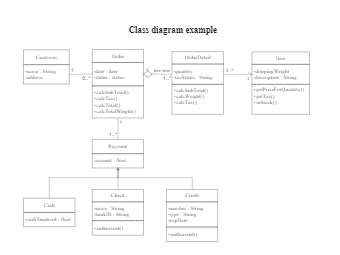This is an example of a uml class diagram.
Class diagram example
203
6
4easy diagrams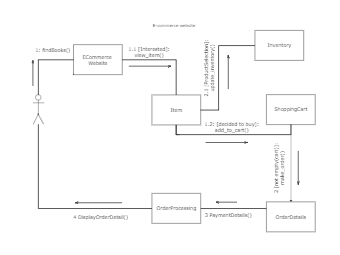This is an example of a UML collaboration diagram.
ecommerce website - UML diagram
24
2
0easy diagrams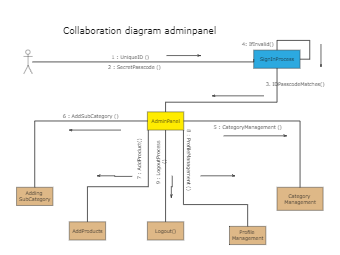11
1
0easy diagrams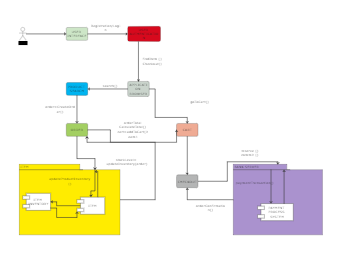This is an example of a UML communication diagram.
Communication diagram example
11
1
1easy diagrams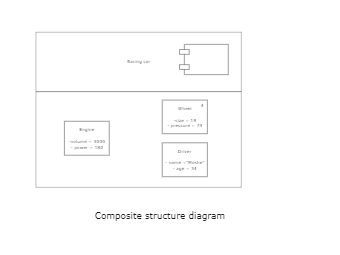This is an example of a composite structure diagram.
Composite structure diagram
3
0
0easy diagrams